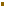1. Big BangCosmology

The principle features of Big Bang cosmology under discussion have been a) t=0", which represents the beginning of time in this cosmology (and thus the age of the universe), and b) the Anthropic Principle (AP), which points to the remarkable conditions placed on the fundamental constants and the laws of nature if the evolution of life in the universe is to be possible.

Science minisummary: Big Bang cosmology.During the decade following the publication of his special theory of relativity, Einstein worked on applying it to a dynamical theory of gravity. His basic insight was to reconceptualize gravity as the curvature of spacetime instead of as a (Newtonian) force in space. Rather than being deflecting from their otherwise linear motion in a Euclidean space with three dimensions, masses would move along geodesics describing the shortest possible path in curved spacetime. Their motion, in turn, would alter the curvature of spacetime, thus giving the field equations General Theory of Relativity (GR) their highly non-linear form aptly described as: spacetime tells mass how to move; mass tells spacetime how to curve.Shortly after the discovery of GR, solutions to Einsteins equations were developed for two distinct classes of problems: i) point masses, which when applied to the solar system led to several key tests of the theory and their eventual confirmation (including the deflection of starlight by the sun and the precession in the perihelion of the orbit of Mercury), and ii) dust, which when eventually applied to the distribution of galaxies and galactic clusters described the universe as expanding in time. During the 1920s, telescopic observations by Edwin Hubble showed that galaxies were indeed receding from us and at a velocity proportional to their distance.In essence, the expansion of the universe had been discovered!

There are in fact three types of expansion possible.i) Closed model: spherical. In one model the universe has the shape of a 3-dimensional sphere of finite size. It expands up to a maximum size, approximately 100 billion years from now, then recontracts, eventually recollapsing to a singularity that mirrors t=0 with infinite temperatures and densities. ii) Open model 1: flat and iii) open model 2: saddle-shaped. Both the flat and saddle-shaped models are infinite in size and expanding in time. In both cases the universe will expand forever and cool indefinitely towards absolute zero.The future of these models is often used to characterize them as freeze (open, both cases) or fry (closed). All three came to be called Big Bang models because they describe the universe as having a finite past life of 10-20 billion years and beginning in an event of infinite temperature and density, and zero volume. Since the age of the universe, t, is calculated as starting here, it is convenient to label it t=0"; technically this event is referred to as an essential singularity.In the 1960s, Stephen Hawking, Roger Penrose, and Robert Geroch proved key theorems which showed that the existence of an essential singularity, t=0, given Einsteins GR, was unavoidable.Contributed by: Dr. Robert Russell